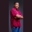Related Tags

swift
communitycreator

# How to create an empty set in SwiftTheodore Kelechukwu Onyejiaku

## Overview

A set is empty if it has no element. In Swift, we can create an empty set very simply.

### Syntax

setName = Set<dataType>()
Syntax for creating an Empty Set in Swift

### Parameters

• setName: This is the name of the empty set that we want to create.
• dataType: This represents the type of data the empty element should have.

### Return value

A set of a specified data type that is empty is returned.

### Example

import Swift

// create empty sets
let intSet = Set<Int>()
let stringSet = Set<String>()
let boolSet = Set<Bool>()
let floatSet = Set<Float>()

// create a set that is not empty
let evenNumbers: Set<Int> = [2, 4, 6, 8, 10]

// print sets
print(intSet)
print(stringSet)
print(boolSet)
print(floatSet)
print(evenNumbers)

// check if they are empty
print(intSet.isEmpty)
print(stringSet.isEmpty)
print(boolSet.isEmpty)
print(floatSet.isEmpty)
print(evenNumbers.isEmpty)
Create an empty set in Swift

### Explanation

• Line 4–7: We create some empty sets.
• Line 10: We create a set that is not empty.
• Line 13–17: We print the sets we created to the console.
• Line 20–24: With the isEmpty method, which returns true if a set is empty, We check if the sets are empty and print the values to the console.

RELATED TAGS

swift
communitycreator

CONTRIBUTORTheodore Kelechukwu Onyejiaku
RELATED COURSES

View all Courses

Keep Exploring

Learn in-demand tech skills in half the time